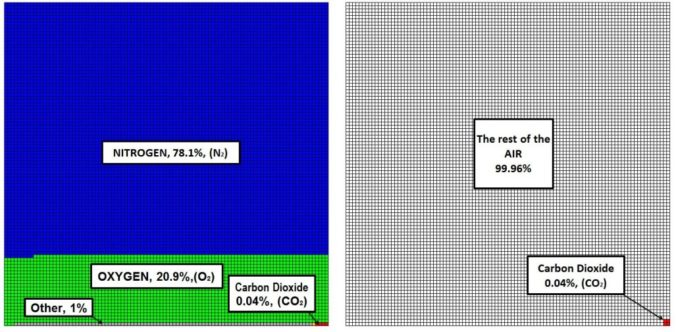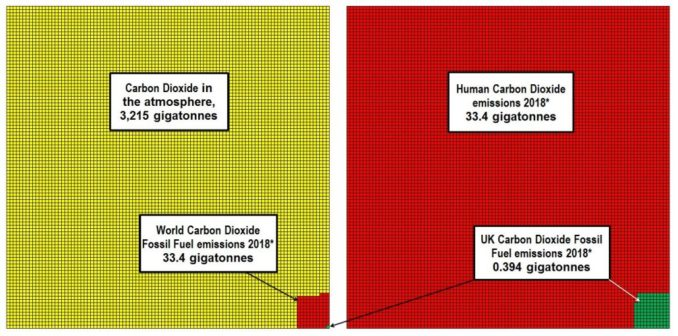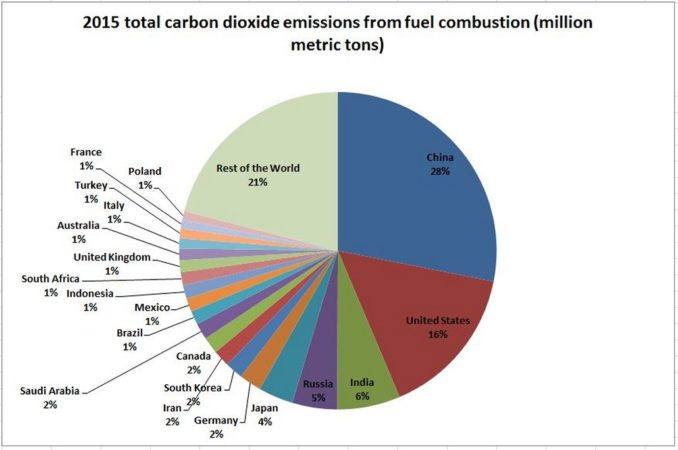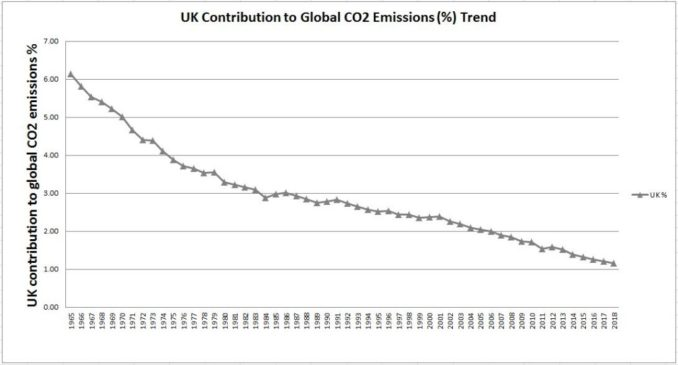# Confronting The Climate Emergency, Part 5

## Magnitude - Diary From The Coal Face - Cllr James Dalton, Holme Valley Parish CouncilThe fourth meeting of the Holme Valley Parish Council Climate Emergency Steering Group [HVPCCESG] took place on 10/7/19. Unfortunately I was unable to attend this rearranged meeting and sent my apologies.

Interesting facts:

• Carbon dioxide is a molecule with a mass number of 44.01. It consists of two oxygen atoms, each with a mass number of 16, and one carbon atom with a mass number of 12. (Carbon also exists as isotopes ‘Carbon 13 and more rarely the unstable isotope Carbon 14 – thus the ‘average’ mass number of Carbon is 12.01)
• Numbers of atoms or molecules are measured in ‘moles’ or ‘mol’. 1 mol of CO2 has a mass of 44.01g and contains 6.022140857 × 1023molecules. 6.022140857 × 1023is Avogadro’s number and is the number of units of one mole of any substance.
• Carbon dioxide is the primary food source of plants from which they obtain carbon: the key constituent of their cellular structures.

I have been pondering on why genuine people are so bought in to the ‘Climate Emergency’.  My son has helpfully been undertaking a ‘Vox pop’ anecdotal survey on what the public know about how big a problem CO2 is. He has been asking people: “How much CO2 is there in the atmosphere?” The result has been revealing – not many people know and many people’s estimates are out by factors of 100 or more!  The answer is approximately 405 parts per million (ppm) or 0.04%. Not very much maybe, but how much is not very much?

How Much CO2?

I then got to pondering: How much CO2 is there in the atmosphere? 0.04% of how much?

The calculation to approximate this is quite easy with a bit of basic maths and physics. (The values derived below will be a slight over approximation of air mass due to no account being taken in the calculation of the volume of land above sea level not occupied by the atmosphere.)

Firstly we can simply consider the weight of the air over a specific area of the earth’s surface, say 1m2 for convenience. Datasets tell us that the average air pressure at the earth’s surface is approximately 101.3 kilopascals (kPa) or 101 300 N/m2 (one Pascal = one Newton per square metre).

Secondly we can estimate the mass of the air by dividing the air weight (101 300 N) by the acceleration due to gravity at the earth’s surface, g, taken as 9.806 m/s2.  (Force = Mass x Acceleration)

Thus, over each square metre of earth, there is approximately 101 300/9.81 = 10 330kg or

wAIR = 10.33 Tonnes/m2.

We can calculate the mass of the whole of the atmosphere by multiplying this figure by the surface area of the earth.

The earth’s diameter varies as it is not a perfect sphere. The average diameter is recorded as 12 742 km, radius = 6 371 000 m

The surface area of a sphere is 4πr2  gives us…

AE = 510.1×1012 m2 for the surface area of the earth.

A simple multiplication gives us the total weight of the atmosphere. However, we must appreciate that although there are approximately 405 parts per million of CO2 in the atmosphere, different molecules have different weights. The mass number of Carbon Dioxide (CO2) is 44.05, with the predominant gases (Nitrogen (N2) and Oxygen (O2)) having lower mass numbers (28 and 32 respectively).  At 0.04% proportion by volume, CO2 constitutes 0.061% of air by mass.

WAIR = wAIR x AE = 510.1×1012 x 10.33  = 5.269×1015 Tonnes  = mass of air in the atmosphere

WCO20.00061 x 5.269×1015  =  3.214×1012 Tonnes  = mass of CO2 in the atmosphere.

3.214×1012 Tonnes (t)  =  3.214×106 Megatonnes (Mt)  =  3 214 Gigatonnes (Gt)

It is a big number, hard to imagine.Burning fossil fuels in the UK adds approximately 0.012% per year to the CO2 already present in the atmosphere

But how much CO2 are humans generating through using fossil fuels? These statistics are published by various government and non-government agencies and researchers. The figure varies slightly depending upon the methods used to calculate, however there is not much divergence and the approximate figure is 33.4 Gt. Of this 33.4 Gt, the UK accounts for approximately 393 Mt, 1.16% of worldwide emissions.CO2 emissions in the short to medium term will be determined by the actions of developing economies, predominantly by China and IndiaAs a contributor to fossil fuel generated CO2, the UK has become less significant over time

In summary, through the use of hydrocarbons and the consequential production of CO2, humans are adding an amount of CO2 to the atmosphere which equates to approximately 1.0% of the amount of CO2 currently in the atmosphere, the UK’s contribution being 0.012% and that of the Holme Valley approximately 0.000005%.

As for the United Kingdom’s and the Holme Valley’s proposed contribution to ‘solving the problem’ of the ‘Climate Emergency’, what it will cost them in taxes, convenience and wellbeing, and for what benefit, if any, they will derive from it… …more next time.

© Councillor James Dalton 2019, DVP – The Direct Democracy Party. dvparty.uk

The Goodnight Vienna Audio file